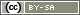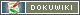# Filter by Text Input

Whether a specific value was selected in a selection or a scale is normally checked. In principle, the process is exactly the same if you want to check to see if a participant wrote something specific in a text input field.

The difference here being that text is compared, rather than figures.

## Check If Something was Entered

Usually, the user just wants to check to see if something was written in a text input field. Or, worded differently for programmers: whether something other than the empty string (no text) was entered. The empty string is written using two single quotation marks ('') or two double quotation marks ("") with nothing in between.

```if (value('AB01_01') != '') {
question('BB01');
}```

The above PHP code works but reacts sensitively to blank spaces: If a participant only enters blank spaces in a text field then this would actually be classified as “not specified”. There is the function `trim()` for this, which removes blanks spaces at the beginning and end. The blank spaces are removed in the filter and then it carries out the comparison:

```if (trim(value('AB01_01')) != '') {
question('BB01');
}```

## Check Minimum Length of Text

`strlen()` is another useful function. It returns the number of characters (length) in a string (text). If we know that any meaningful data must be made up of at least 4 characters then the length of the string can also be checked. `trim()` is used here again so that any blank spaces do not cause any wrong decision-making.

```if (strlen(trim(value('AB01_01'))) > 3) {
question('BB01');
}```

Or more legibly (in case the overview is lost with so many brackets):

```\$input = value('AB01_01');  // participant's input
\$withoutspaces = trim(\$input);   // remove blank spaces at beginning and end
\$length = strlen(\$withoutspaces);  // determine length
if (\$length > 3) {
question('BB01');
}```

## Compare Text

If whether the participant gave the correct answer should be checked, this works by using the comparison operators `==` (equal) and `!=` (not equal) – as with numbers. The following filter should check whether the participant watched a test video. The text video shows a word which should be entered in the input field. If the participant enters the wrong word, the page with the test video is displayed again.

```if (value('AB01_01') != 'cat') {
repeatPage();
}```

If there should not be any fussiness between upper and lower case, writing `strtolower()` in lower case before comparison converts the participant's input.

```if (strtolower(value('AB01_01')) != 'cat') {
repeatPage();
}```

Blank spaces at the beginning/end can be removed once again by using the familiar `trim()`:

```if (strtolower(trim(value('AB01_01'))) != 'cat') {
repeatPage();
}```

## Numbers in the Input

PHP is not fussy when it comes to differentiating between text and numbers. If you want to check if the participant gave a number greater than 10 in the input field `AB01_01`, this can be done as follows:

```if (value('AB01_01') > 10) {
question('BC02');
}```

The input field should, however, then only be set to allow the participant to enter digits (see Check Responses: Entering Numbers). If this cannot be set or is not desired, `is_numeric()` can be used to find out if the particpant entered a number.

The following filter is only effective if the participant gave a number and this number is greater than 10.

```\$input = value('AB01_01');
if (is_numeric(\$input) and (\$input > 10)) {
question('BC02');
}```

Likewise, PHP can be instructed to convert the participant's input into a number as best as possible. Therefore, “5b” becomes `5`. The conversion takes place with the function `intval()` or with the prefix `(int)` (type casting).

```\$input = intval(value('AB01_01'));
if (\$input > 10) {
question('BC02');
}```
```\$input = (int)value('AB01_01');
if (\$input > 10) {
question('BC02');
}```

Take care: Decimal places are cut off when using this solution. If you want to work with decimals, you have to first of all make sure that a period is used as the decimal separator (if necessary, set a text input that only allows decimals in SoSci Survey) and then carry out the conversion into a number using the prefix `(float)`.